Next: Upwind differencing Up: The wave equation Previous: The Lax scheme

## The Crank-Nicholson scheme

The Crank-Nicholson implicit scheme for solving the diffusion equation (see Sect. 6.6) can be adapted to solve the advection equation. Thus, taking the average of the right-hand side of Eq. (234) between the beginning and end of the time-step, we obtain the differencing scheme written below: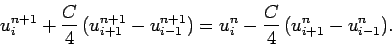(246)

A von Neumann stability analysis of the above scheme yields the following expression for the amplification factor: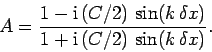(247)

Note that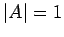for all values of, irrespective of the value of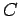. This implies that the Crank-Nicholson implicit scheme is not subject to the CFL constraint,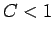(since there is no value offor which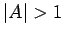). Moreover, there is no spurious decay in the Fourier harmonics of the solution (since). Hence, unlike the Lax scheme, we would not expect the Crank-Nicholson scheme to introduce strong numerical dispersion into the advection problem.

Listed below is a routine which solves the 1-d advection equation via the Crank-Nicholson method.

// Advect1D.cpp

// Function to evolve advection equation in 1-d:

//  du / dt + v du / dx = 0  for  xl <= x <= xh

//  u = 0  at x=xl and x=xh

// Array u assumed to be of extent N+2.

// Now, ith element of array corresponds to

//  x_i = xl + i * dx    i=0,N+1

// Here, dx = (xh - xl) / (N+1) is grid spacing.

// Function evolves u by single time-step.

// C = v dt / dx, where dt is time-step.

// Uses Crank-Nicholson scheme.

#include <blitz/array.h>

using namespace blitz;

void Tridiagonal (Array<double,1> a, Array<double,1> b, Array<double,1> c,
Array<double,1> w, Array<double,1>& u);

void Advect1D (Array<double,1>& u, double C)
{
// Find N. Declare local arrays.
int N = u.extent(0) - 2;
Array<double,1> a(N+2), b(N+2), c(N+2), w(N+2);

// Initialize tridiagonal matrix
for (int i = 2; i <= N;   i++) a(i) = - 0.25 * C;
for (int i = 1; i <= N; i++) b(i) = 1.;
for (int i = 1; i <= N-1; i++) c(i) = + 0.25 * C;

// Initialize right-hand side vector
for (int i = 1; i <= N; i++)
w(i) = u(i) - 0.25 * C * (u(i+1) - u(i-1));

// Invert tridiagonal matrix equation
Tridiagonal (a, b, c, w, u);

// Calculate i=0 and i=N+1 values
u(0) = 0.;
u(N+1) = 0.;
}


Figure 75 shows an example calculation which uses the above routine to advect a Gaussian pulse. The initial condition is as specified in Eq. (244), and the calculation is performed with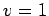,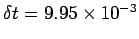, and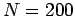. Note that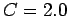for these parameters. It can be seen that the pulse propagates at (almost) the correct speed, and maintains approximately the same shape. Clearly, the performance of the Crank-Nicholson scheme is vastly superior to that of the Lax scheme.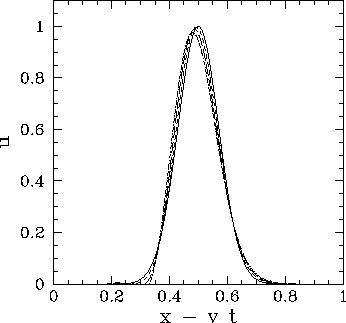Next: Upwind differencing Up: The wave equation Previous: The Lax scheme
Richard Fitzpatrick 2006-03-29